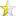### C++

Jun 9, 2012 (last update: Jun 9, 2014)

# Integer overflowOverflow is a phenomenon where operations on 2 numbers exceeds the maximum (or goes below the minimum) value the data type can have. Usually it is thought that integral types are very large and people don't take into account the fact that sum of two numbers can be larger than the range. But in things like scientific and mathematical computation, this can happen. For example, an unhandled arithmetic overflow in the engine steering software was the primary cause of the crash of the maiden flight of the Ariane 5 rocket. The software had been considered bug-free since it had been used in many previous flights; but those used smaller rockets which generated smaller accelerations than Ariane 5's.This article will tell how this problem can be tackled.

In this article, we will only deal with integral types (and not with types like float and double)

In order to understand how to tackle this problem we will first know how numbers are stored.

If the size of a data type is n bytes, it can store 28n different values. This is called the data type's range.
If size of an unsigned data type is n bytes, it ranges from 0 to 28n-1
If size of a signed data type is n bytes, it ranges from -28n-1 to 28n-1-1
So, a short(usually 2 bytes) ranges from -32768 to 32767 and an unsigned short ranges from 0 to 65535

Consider a short variable having a value of 250.
It is stored int the computer like this (in binary format)
00000000 11111010

Complement of a number is a number with its bits toggled. It is denoted by ~
For eg. ~250 is 11111111 00000101

Negative numbers are stored using 2's complement system. According to this system, -n=~n+1
-250 is stored as 11111111 00000110
http://stackoverflow.com/questions/1049722/what-is-2s-complement

10000000 00000000 (-32768) has no positive counterpart. Its negative is the number itself (try -n=~n+1)

11100010 01110101 will be read as 57973 if data type is unsigned while it will be read as -7563 if data type is signed. If you add 65536 (which is the range) to -7563, you get 57973.

Overflow:
Consider a data type var_t of 1 byte (range is 256):
signed var_t a,b;
unsigned var_t c,d;

If c is 200(11001000) and d is 100(01100100), c+d is 300(00000001 00101100), which is more than the max value 255(11111111). 00000001 00101100 is more than a byte, so the higher byte will be rejected and c+d will be read as 44. So, 200+100=44! This is absurd! (Note that 44=300-256). This is an example of an unsigned overflow, where the value couldn't be stored in the available no. of bytes. In such overflows, the result is moduloed by range (here, 256).

If a is 100(01100100) and b is 50(00110010), a+b is 150(10010110), which is more than the max value 127. Instead, a+b will be read as -106 (note that -106=150-256). This is an example of a signed overflow, where result is moduloed by range(here, 256).

### Detecting overflow:

Division and modulo can never generate an overflow.

Overflow can only occur when sign of numbers being added is the same (which will always be the case in unsigned numbers)
signed overflow can be easily detected by seeing that its sign is opposite to that of the operands.

Let us analyze overflow in unsigned integer addition.

Consider 2 variables a and b of a data type with size n and range R.
Let + be actual mathematical addition and a\$b be the addition that the computer does.

If a+b<=R-1, a\$b=a+b
As a and b are unsigned, a\$b is more or equal to than both a and b.

If a+b>=R a\$b=a+b-R
as R is more than both a and b, a-R and b-R are negative
So, a+b-R<a and a+b-R<b
Therefore, a\$b is less than both a and b.

This difference can be used to detect unsigned addition overflow. a-b can be treated as a+(-b) hence subtraction can be taken care of in the same way.

Multiplication overflow: There are two ways to detect an overflow:

1. if a*b>max, then a>max/b (max is R-1 if unsigned and R/2-1 if signed).
2. Let there be a data type of size n and range R called var_t and a data type of size 2n called var2_t.
Let there be 2 variables of var_t called a and b. Range of var2_t will be R*R, which will always be more than the product of a and b. hence if var2_t(a)*var2_t(b)>R overflow has happened.

Truncation: This happens when a shorter is assigned from a longer variable. For eg, `short a;long b=70000;a=b;` Only the lower bits are copied and the value's meaning is translated.
`short a;int b=57973;a=b;` will also show this behaviour become -7563.
Similar behaviour will be shown if int is replaced by unsigned short.

Type conversion: consider `unsigned int a=4294967290;int b=-6; return (a==b);` This returns 1.
Whenever an operation is performed between an unsigned and a signed variable of the same type, operands are converted to unsigned.
Whenever an operation is performed between a long type and a short type, operands are converted to the long type.
The above code returned 1 as a and b were converted to unsigned int and then compared.
If we used __int64 (a 64-bit type) instead of unsigned int and 18446744073709551610 instead of 4294967290, the result would have been the same.

Type promotion: Whenever an operation is performed on two variables of a type shorter than int, the type of both variables is converted to int. For eg. `short a=32000,b=32000;cout<<a+b<<endl;` would display 64000, which is more than the max value of short. The reason is that a and b were converted to int and a+b would return an int, which can have a value of 64000.

### Libraries:

Microsoft Visual C++ 2010 has a header file safeint.h which has functions like safeadd,safesubtract,etc. It is a templated header file (and hence header only).